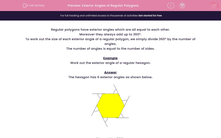# Exterior Angles of Regular Polygons

In this worksheet, students calculate the exterior angle of regular polygons.Key stage:  KS 2

Curriculum topic:   Maths and Numerical Reasoning

Curriculum subtopic:   2D Shapes: Circles, Angles and Bearings

Difficulty level:#### Worksheet Overview

Regular polygons have exterior angles which are all equal to each other.

Moreover they always add up to 360º.

To work out the size of each exterior angle of a regular polygon, we simply divide 360º by the number of angles.

The number of angles is equal to the number of sides.

Example

Work out the exterior angle of a regular hexagon.

The hexagon has 6 exterior angles as shown below.